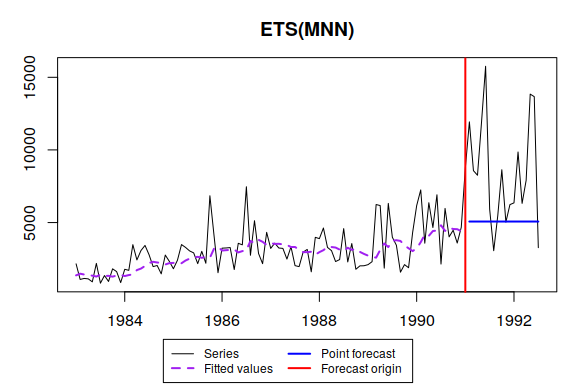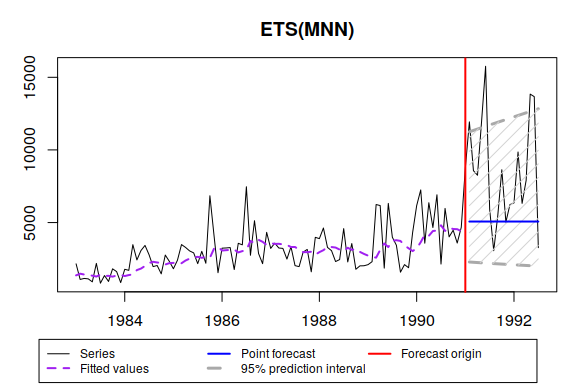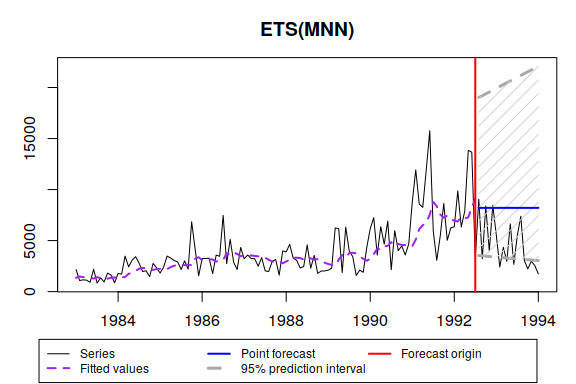# es() - Exponential Smoothing

#### 2019-04-25

es() is a part of smooth package. It allows constructing Exponential Smoothing (also known as ETS), selecting the most appropriate one among 30 possible ones, including exogenous variables and many more.

In this vignette we will use data from Mcomp package, so it is advised to install it. We also use some of the functions of the greybox package.

require(smooth)
require(greybox)
require(Mcomp)

You may note that Mcomp depends on forecast package and if you load both forecast and smooth, then you will have a message that forecast() function is masked from the environment. There is nothing to be worried about - smooth uses this function for consistency purposes and has exactly the same original forecast() as in the forecast package. The inclusion of this function in smooth was done only in order not to include forecast in dependencies of the package.

The simplest call of this function is:

es(M3$N2457$x, h=18, holdout=TRUE, silent=FALSE)
## Forming the pool of models based on... ANN, ANA, AAN, Estimation progress:    100%... Done!
## Time elapsed: 0.6 seconds
## Model estimated: ETS(MNN)
## Persistence vector g:
## alpha
## 0.145
## Initial values were optimised.
## 3 parameters were estimated in the process
## Residuals standard deviation: 0.407
## Cost function type: MSE; Cost function value: 0.165
##
## Information criteria:
##      AIC     AICc      BIC     BICc
## 1645.978 1646.236 1653.702 1654.292
## Forecast errors:
## MPE: 26.3%; sCE: -1919.1%; Bias: 86.9%; MAPE: 39.8%
## MASE: 2.944; sMAE: 120.1%; sMSE: 242.7%; RelMAE: 1.258; RelRMSE: 1.367In this case function uses branch and bound algorithm to form a pool of models to check and after that constructs a model with the lowest information criterion. As we can see, it also produces an output with brief information about the model, which contains:

1. How much time was elapsed for the model construction;
2. What type of ETS was selected;
3. Values of persistence vector (smoothing parameters);
4. What type of initialisation was used;
5. How many parameters were estimated (standard deviation is included);
6. Standard deviation of residuals. The model has multiplicative error term, so as a result the standard deviation is small.
7. Cost function type and the value of that cost function;
8. Information criteria for this model;
9. Forecast errors (because we have set holdout=TRUE).

The function has also produced a graph with actuals, fitted values and point forecasts.

If we need prediction intervals, then we run:

es(M3$N2457$x, h=18, holdout=TRUE, intervals=TRUE, silent=FALSE)
## Forming the pool of models based on... ANN, ANA, AAN, Estimation progress:    100%... Done!
## Time elapsed: 0.4 seconds
## Model estimated: ETS(MNN)
## Persistence vector g:
## alpha
## 0.145
## Initial values were optimised.
## 3 parameters were estimated in the process
## Residuals standard deviation: 0.407
## Cost function type: MSE; Cost function value: 0.165
##
## Information criteria:
##      AIC     AICc      BIC     BICc
## 1645.978 1646.236 1653.702 1654.292
## 95% parametric prediction intervals were constructed
## 72% of values are in the prediction interval
## Forecast errors:
## MPE: 26.3%; sCE: -1919.1%; Bias: 86.9%; MAPE: 39.8%
## MASE: 2.944; sMAE: 120.1%; sMSE: 242.7%; RelMAE: 1.258; RelRMSE: 1.367Due to multiplicative nature of error term in the model, the intervals are asymmetric. This is the expected behaviour. The other thing to note is that the output now also provides the theoretical width of prediction intervals and its actual coverage.

If we save the model (and let’s say we want it to work silently):

ourModel <- es(M3$N2457$x, h=18, holdout=TRUE, silent="all")

we can then reuse it for different purposes:

es(M3$N2457$x, model=ourModel, h=18, holdout=FALSE, intervals="np", level=0.93)
## Time elapsed: 0.05 seconds
## Model estimated: ETS(MNN)
## Persistence vector g:
## alpha
## 0.145
## Initial values were provided by user.
## 1 parameter was estimated in the process
## 2 parameters were provided
## Residuals standard deviation: 0.429
## Cost function type: MSE; Cost function value: 0.184
##
## Information criteria:
##      AIC     AICc      BIC     BICc
## 1994.861 1994.897 1997.606 1997.690
## 93% nonparametric prediction intervals were constructed

We can also extract the type of model in order to reuse it later:

modelType(ourModel)
##  "MNN"

This handy function, by the way, also works with ets() from forecast package.

We can then use persistence or initials only from the model to construct the other one:

es(M3$N2457$x, model=modelType(ourModel), h=18, holdout=FALSE, initial=ourModel$initial, silent="graph") ## Time elapsed: 0.02 seconds ## Model estimated: ETS(MNN) ## Persistence vector g: ## alpha ## 0.151 ## Initial values were provided by user. ## 2 parameters were estimated in the process ## 1 parameter was provided ## Residuals standard deviation: 0.429 ## Cost function type: MSE; Cost function value: 0.184 ## ## Information criteria: ## AIC AICc BIC BICc ## 1996.845 1996.952 2002.334 2002.589 es(M3$N2457$x, model=modelType(ourModel), h=18, holdout=FALSE, persistence=ourModel$persistence, silent="graph")
## Time elapsed: 0.02 seconds
## Model estimated: ETS(MNN)
## Persistence vector g:
## alpha
## 0.145
## Initial values were optimised.
## 2 parameters were estimated in the process
## 1 parameter was provided
## Residuals standard deviation: 0.429
## Cost function type: MSE; Cost function value: 0.184
##
## Information criteria:
##      AIC     AICc      BIC     BICc
## 1996.861 1996.968 2002.351 2002.605

or provide some arbitrary values:

es(M3$N2457$x, model=modelType(ourModel), h=18, holdout=FALSE, initial=1500, silent="graph")
## Time elapsed: 0.02 seconds
## Model estimated: ETS(MNN)
## Persistence vector g:
## alpha
##  0.15
## Initial values were provided by user.
## 2 parameters were estimated in the process
## 1 parameter was provided
## Residuals standard deviation: 0.429
## Cost function type: MSE; Cost function value: 0.184
##
## Information criteria:
##      AIC     AICc      BIC     BICc
## 1997.028 1997.136 2002.518 2002.773

Using some other parameters may lead to completely different model and forecasts:

es(M3$N2457$x, h=18, holdout=TRUE, cfType="aTMSE", bounds="a", ic="BIC", intervals=TRUE)
## Time elapsed: 0.43 seconds
## Model estimated: ETS(ANN)
## Persistence vector g:
## alpha
##  0.08
## Initial values were optimised.
## 3 parameters were estimated in the process
## Residuals standard deviation: 1444.05
## Cost function type: aTMSE; Cost function value: 39565651.9
##
## Information criteria:
##      AIC     AICc      BIC     BICc
## 1974.076 1974.736 1985.865 1986.455
## 95% parametric prediction intervals were constructed
## 44% of values are in the prediction interval
## Forecast errors:
## MPE: 33.4%; sCE: -2196.8%; Bias: 90.4%; MAPE: 43.4%
## MASE: 3.235; sMAE: 132%; sMSE: 278%; RelMAE: 1.382; RelRMSE: 1.463

You can play around with all the available parameters to see what’s their effect on final model.

In order to combine forecasts we need to use “C” letter:

es(M3$N2457$x, model="CCN", h=18, holdout=TRUE, silent="graph")
## Estimation progress:    10%20%30%40%50%60%70%80%90%100%... Done!
## Time elapsed: 0.69 seconds
## Model estimated: ETS(CCN)
## Initial values were optimised.
## Residuals standard deviation: 1409.001
## Cost function type: MSE
##
## Information criteria:
## (combined values)
##      AIC     AICc      BIC     BICc
## 1647.275 1647.545 1654.044 1654.524
## Forecast errors:
## MPE: 26.7%; sCE: -1936.1%; Bias: 87.4%; MAPE: 40%
## MASE: 2.963; sMAE: 120.9%; sMSE: 245%; RelMAE: 1.266; RelRMSE: 1.373

Model selection from a specified pool and forecasts combination are called using respectively:

es(M3$N2457$x, model=c("ANN","AAN","AAdN","ANA","AAA","AAdA"), h=18, holdout=TRUE, silent="graph")
## Estimation progress:    17%33%50%67%83%100%... Done!
## Time elapsed: 0.76 seconds
## Model estimated: ETS(ANN)
## Persistence vector g:
## alpha
## 0.158
## Initial values were optimised.
## 3 parameters were estimated in the process
## Residuals standard deviation: 1416.935
## Cost function type: MSE; Cost function value: 2007704.532
##
## Information criteria:
##      AIC     AICc      BIC     BICc
## 1688.987 1689.245 1696.711 1697.301
## Forecast errors:
## MPE: 25.3%; sCE: -1880.4%; Bias: 86%; MAPE: 39.4%
## MASE: 2.909; sMAE: 118.7%; sMSE: 238.1%; RelMAE: 1.243; RelRMSE: 1.354
es(M3$N2457$x, model=c("CCC","ANN","AAN","AAdN","ANA","AAA","AAdA"), h=18, holdout=TRUE, silent="graph")
## Estimation progress:    17%33%50%67%83%100%... Done!
## Time elapsed: 0.75 seconds
## Model estimated: ETS(CCC)
## Initial values were optimised.
## Residuals standard deviation: 1386.692
## Cost function type: MSE
##
## Information criteria:
## (combined values)
##      AIC     AICc      BIC     BICc
## 1689.848 1690.146 1696.984 1697.488
## Forecast errors:
## MPE: 17.1%; sCE: -1568.3%; Bias: 77.7%; MAPE: 37.3%
## MASE: 2.658; sMAE: 108.4%; sMSE: 206.7%; RelMAE: 1.135; RelRMSE: 1.261

Now let’s introduce some artificial exogenous variables:

x <- cbind(rnorm(length(M3$N2457$x),50,3),rnorm(length(M3$N2457$x),100,7))

and fit a model with all the exogenous first:

es(M3$N2457$x, model="ZZZ", h=18, holdout=TRUE, xreg=x)
## Time elapsed: 0.73 seconds
## Model estimated: ETSX(MNN)
## Persistence vector g:
## alpha
## 0.148
## Initial values were optimised.
## 5 parameters were estimated in the process
## Residuals standard deviation: 0.403
## Xreg coefficients were estimated in a normal style
## Cost function type: MSE; Cost function value: 0.163
##
## Information criteria:
##      AIC     AICc      BIC     BICc
## 1648.352 1649.012 1661.226 1662.734
## Forecast errors:
## MPE: 23.8%; sCE: -1807.1%; Bias: 84.9%; MAPE: 38%
## MASE: 2.821; sMAE: 115.1%; sMSE: 230.1%; RelMAE: 1.205; RelRMSE: 1.331

or construct a model with selected exogenous (based on IC):

es(M3$N2457$x, model="ZZZ", h=18, holdout=TRUE, xreg=x, xregDo="select")
## Time elapsed: 0.41 seconds
## Model estimated: ETS(MNN)
## Persistence vector g:
## alpha
## 0.145
## Initial values were optimised.
## 3 parameters were estimated in the process
## Residuals standard deviation: 0.407
## Cost function type: MSE; Cost function value: 0.165
##
## Information criteria:
##      AIC     AICc      BIC     BICc
## 1645.978 1646.236 1653.702 1654.292
## Forecast errors:
## MPE: 26.3%; sCE: -1919.1%; Bias: 86.9%; MAPE: 39.8%
## MASE: 2.944; sMAE: 120.1%; sMSE: 242.7%; RelMAE: 1.258; RelRMSE: 1.367

or the one with the updated xreg:

ourModel <- es(M3$N2457$x, model="ZZZ", h=18, holdout=TRUE, xreg=x, updateX=TRUE)

If we want to check if lagged x can be used for forecasting purposes, we can use xregExpander() function from greybox package:

es(M3$N2457$x, model="ZZZ", h=18, holdout=TRUE, xreg=xregExpander(x), xregDo="select")
## Time elapsed: 1.38 seconds
## Model estimated: ETSX(MNN)
## Persistence vector g:
## alpha
## 0.147
## Initial values were optimised.
## 4 parameters were estimated in the process
## Residuals standard deviation: 0.403
## Xreg coefficients were estimated in a normal style
## Cost function type: MSE; Cost function value: 0.163
##
## Information criteria:
##      AIC     AICc      BIC     BICc
## 1646.458 1646.893 1656.757 1657.752
## Forecast errors:
## MPE: 27.5%; sCE: -1991.7%; Bias: 88%; MAPE: 40.9%
## MASE: 3.041; sMAE: 124.1%; sMSE: 259.2%; RelMAE: 1.299; RelRMSE: 1.412

If we are confused about the type of estimated model, the function formula() will help us:

formula(ourModel)
##  "y[t] = l[t-1] * exp(a1[t-1] * x1[t] + a2[t-1] * x2[t]) * e[t]"

A feature available since 2.1.0 is fitting ets() model and then using its parameters in es():

etsModel <- forecast::ets(M3$N2457$x)
esModel <- es(M3$N2457$x, model=etsModel, h=18)

The point forecasts in the majority of cases should the same, but the prediction intervals may be different (especially if error term is multiplicative):

forecast(etsModel,h=18,level=0.95)
##          Point Forecast       Lo 95    Hi 95
## Aug 1992       8523.456   853.30277 16193.61
## Sep 1992       8563.040   719.69262 16406.39
## Oct 1992       8602.625   587.42532 16617.82
## Nov 1992       8642.209   456.39433 16828.02
## Dec 1992       8681.794   326.50223 17037.09
## Jan 1993       8721.379   197.65965 17245.10
## Feb 1993       8760.963    69.78442 17452.14
## Mar 1993       8800.548   -57.19924 17658.29
## Apr 1993       8840.132  -183.36139 17863.63
## May 1993       8879.717  -308.76695 18068.20
## Jun 1993       8919.302  -433.47621 18272.08
## Jul 1993       8958.886  -557.54529 18475.32
## Aug 1993       8998.471  -681.02653 18677.97
## Sep 1993       9038.055  -803.96882 18880.08
## Oct 1993       9077.640  -926.41794 19081.70
## Nov 1993       9117.225 -1048.41679 19282.87
## Dec 1993       9156.809 -1170.00570 19483.62
## Jan 1994       9196.394 -1291.22258 19684.01
forecast(esModel,h=18,level=0.95)
##          Point forecast Lower bound (2.5%) Upper bound (97.5%)
## Aug 1992       9352.900           3661.607            19667.82
## Sep 1992       9534.040           3664.498            20407.03
## Oct 1992       9765.247           3662.563            21211.15
## Nov 1992       9973.668           3721.224            21972.67
## Dec 1992      10192.885           3751.618            22487.85
## Jan 1993      10398.648           3752.026            23342.72
## Feb 1993      10625.812           3806.078            24186.38
## Mar 1993      10829.256           3811.182            24978.04
## Apr 1993      11061.514           3818.255            25816.54
## May 1993      11290.470           3844.685            26350.27
## Jun 1993      11524.842           3866.250            27321.64
## Jul 1993      11779.648           3913.325            28129.69
## Aug 1993      11989.252           3901.180            28933.17
## Sep 1993      12288.172           3959.410            29923.20
## Oct 1993      12530.298           3972.137            30594.77
## Nov 1993      12774.302           4017.697            31655.47
## Dec 1993      13038.866           4053.363            32484.63
## Jan 1994      13313.902           4086.671            33466.95

Finally, if you work with M or M3 data, and need to test a function on a specific time series, you can use the following simplified call:

es(M3\$N2457, intervals=TRUE, silent=FALSE)
## Forming the pool of models based on... ANN, ANA, AAN, Estimation progress:    100%... Done!
## Time elapsed: 0.41 seconds
## Model estimated: ETS(MNN)
## Persistence vector g:
## alpha
## 0.151
## Initial values were optimised.
## 3 parameters were estimated in the process
## Residuals standard deviation: 0.429
## Cost function type: MSE; Cost function value: 0.184
##
## Information criteria:
##      AIC     AICc      BIC     BICc
## 1998.844 1999.061 2007.079 2007.592
## 95% parametric prediction intervals were constructed
## 50% of values are in the prediction interval
## Forecast errors:
## MPE: -127.6%; sCE: 1618.3%; Bias: -92.4%; MAPE: 129.2%
## MASE: 2.278; sMAE: 93.4%; sMSE: 115.4%; RelMAE: 1.895; RelRMSE: 1.586This command has taken the data, split it into in-sample and holdout and produced the forecast of appropriate length to the holdout.